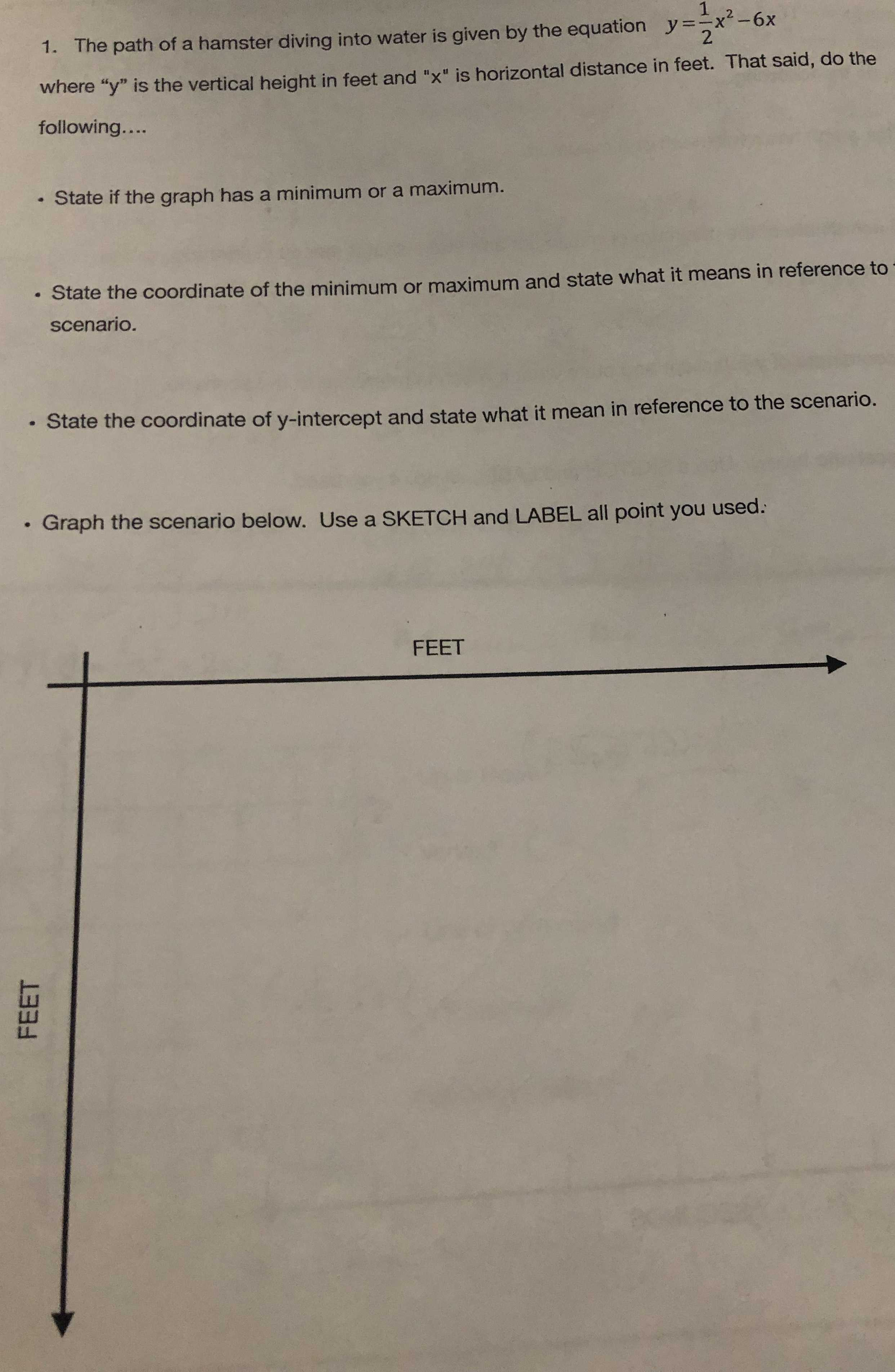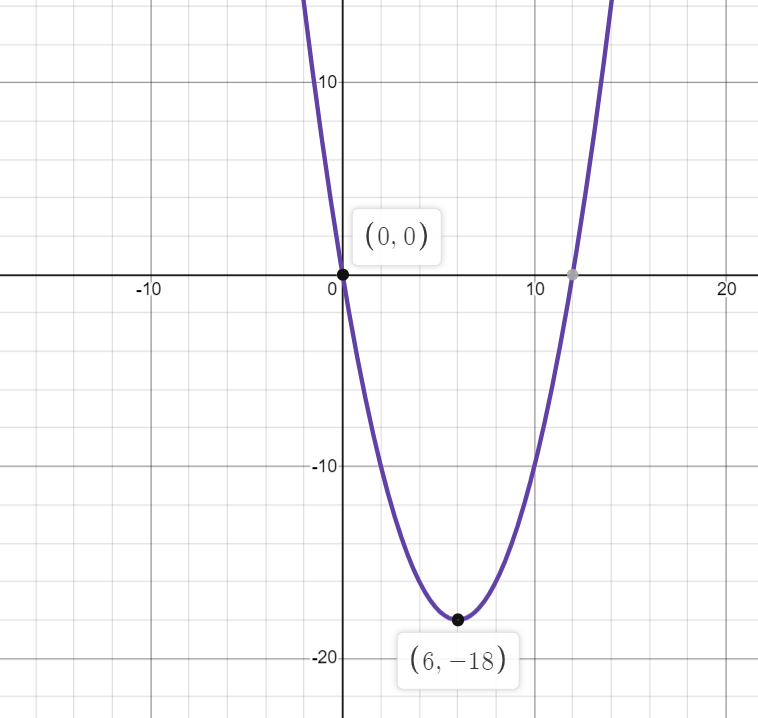### ¿Todavía tienes preguntas de matemáticas?

Pregunte a nuestros tutores expertos
Algebra
Pregunta1. The path of a hamster diving into water is given by the equation $$y = \frac { 1 } { 2 } x ^ { 2 } - 6 x$$

where " $$y$$ " is the vertical height in feet and " $$x$$ " is horizontal distance in feet. That said, do the following....

- State if the graph has a minimum or a maximum.

State the coordinate of the minimum or maximum and state what it means in reference to scenario.

- State the coordinate of $$y$$ -intercept and state what it mean in reference to the scenario.

- Graph the scenario below. Use a SKETCH and LABEL all point you used: